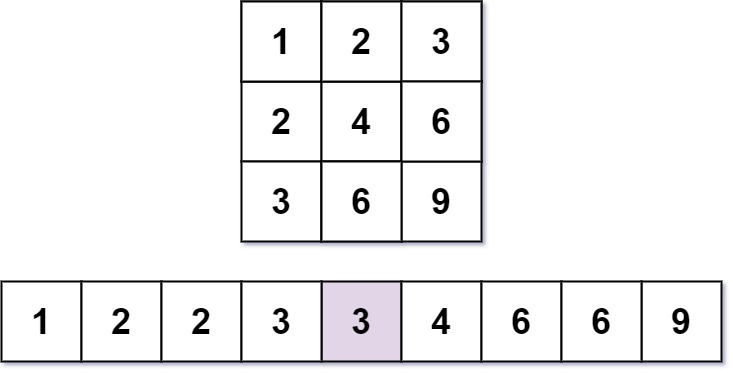# Kth Smallest Number in Multiplication Table Leetcode Solution

Difficulty Level Hard

## Problem Statement

The Kth Smallest Number in Multiplication Table Solution – states that you are given the multiplication table matrix of size m x n, where matrix[i][j] = i*j (1 indexed).

For the given three integers m,n and k, we need to find the kth smallest element in the m x n multiplication table.

## Example:`Input: m = 3, n = 3, k = 5`
`Output: 3`

Explanation:

• The elements of 3 x 3 multiplication table in sorted form are: [1, 2, 2, 3, 3, 4, 6, 6, 9].
• The 5th smallest number is 3.

`Input: m = 2, n = 3, k = 6`
`Output: 6`

Explanation:

• The elements of 2 x 3 multiplication table in sorted form are: [1, 2, 2, 3, 4, 6].
• The kth smallest number is: 6

## Approach

### Idea:

1. The main idea to solve this problem efficiently is Binary Search.
2. Consider the lower bound (l) for binary search as the minimum element of the matrix which is matrix.
3. And, Consider the upper bound (r) for binary search as the maximum element of the matrix which is matrix[m][n].
4. Now, the kth smallest number in the multiplication table is equal to the smallest number in the range [l,r] which has k-1 smaller elements than self.
5. We can perform Binary Search. For the current position mid, Let cnt denotes the number of elements in the matrix that are smaller than mid.
• If cnt< k, there are less than k numbers that are less than or equal to mid in the table. So mid or any integer lower than it can’t be our answer. So eliminate search space lower than mid by doing l = mid + 1.
• If cnt >= k, there are at least k numbers (maybe more) that are less than or equal to mid in the table. So mid is the possible valid solution. But there can be a smaller number than mid as well as cnt >= k. So mark mid as possible answer and make r = mid – 1.
• Finally, we return the answer which is the required kth smallest element in the table.
6. Now, to find a number of strictly smaller elements than mid, we need to iterate for all rows. Our answer will be incremented by the floor value obtained as a result of the division of mid by row number for each row. Note that the value obtained for each row must be at most n(number of columns of the matrix).

## Dry Run With Explanation For Sample Input 1:## Code

### Kth Smallest Number in the Multiplication Table Leetcode C++ Solution:

```class Solution {
public:
int findKthNumber(int m, int n, int k) {
int l = 1,r = m*n,ans = r;
while(l<=r){
int mid = (l+r)/2,cnt = 0;
for(int i=1;i<=m;i++){
cnt += min(n,mid/i);
}
if(cnt>=k){
ans = mid;
r = mid - 1;
}
else{
l = mid + 1;
}
}
return ans;
}
};```

### Kth Smallest Number in the Multiplication Table Leetcode Java Solution:

```class Solution {
public int findKthNumber(int m, int n, int k) {
int l = 1,r = m*n,ans = r;
while(l<=r){
int mid = (l+r)/2,cnt = 0;
for(int i=1;i<=m;i++){
cnt += Math.min(n,mid/i);
}
if(cnt>=k){
ans = mid;
r = mid - 1;
}
else{
l = mid + 1;
}
}
return ans;
}
}```

## Complexity Analysis for Kth Smallest Number in the Multiplication Table Leetcode Solution

### Time Complexity

The time complexity of the above code is O(m*log(m*n)), where m = number of rows of matrix and n = number of columns of the matrix.

Note that each time search space is reduced to half because of the binary search hence, the log(m*n) factor comes. And, for each time, we need to iterate exactly m times. Hence O(m*log(m*n)).

### Space Complexity

The space complexity of the above code O(1) since we’re using constant extra space.

Translate »